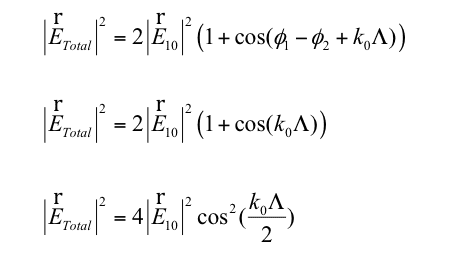# Why does cos(phi1- phi 2 +kX)=cos(kx)??

• I
In the case of two fields interfering with each other when calculating the total electric field, cos (phi1-phi2 + kx) = cos( kx) where kx is the path difference between the two fields.

How does cos (phi1-phi2 +kx)=cos(kx) Isit just algebra?blue_leaf77
Homework Helper
Is it said anything about ##\phi_1-\phi_2##? Those equality can be true if ##\phi_1-\phi_2## is a multiple of ##2\pi##.

Earlier on a general interference between two waves is obtained and it is assumed that they have the same phase velocity and so phi1=phi2, and the function collapses. Perhaps this is the case here?

blue_leaf77
Homework Helper
Is ##\phi = \omega t ##?

I dont think it is, as wt is mentioned seperately when phi is mentioned. Phi is mentioned when defining the amplitude of the wave Eo= |Eo|e^iphi{Eo}, where {Eo} is the unit vector.

vanhees71
$$\phi=A_0 \cos(\omega t-k x+\phi_1)+A_1 \cos(\omega t-k x+\phi_2)?$$